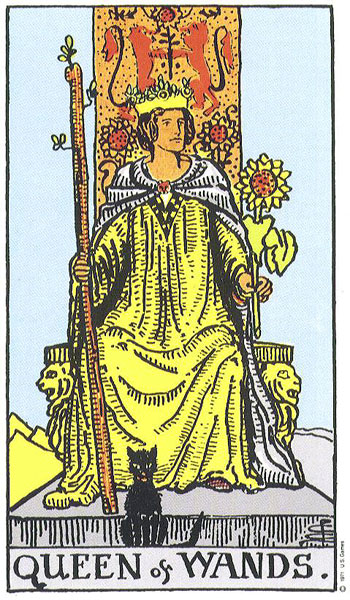## people with a 24 (Queen of Wands) life lesson

### July 5, 2000people with a 24 life lesson:Pat Boone born June 1st, 1934

June 1st, 1934

6 + 1 +1+9+3+4 = 24 = his life lesson = what he is here to learn = Dominance.  Clean living.  Wholesome.  Fit & trim.  Devotion.  Vows.  Pledge.  Commitment.  Purity.  Nutrition.  Nourishment.  Milk.  Clean diet.  Whole foods.  Organic foods.  Natural living.  Natural diet.  Bodily purity.  Nutrients.  Vitamins.  Supplements.  Fortified.  Self-contained.  Abstinence.  Celibacy.  Chastity.  Virgin.  Pure.  Demure.  Modesty.  Short hair.  Clean cut.

Jane Goodall
born April 3rd, 1934                        4 + 3 +1+9+3+4 = 24

Tom Smothers
born February 2nd, 1937                 2 + 2 +1+9+3+7= 24

Helen Thomas
born August 4th, 1920                     8 + 4 +1+9+2+0 = 24

Dave Thomas
born July 2nd, 1932                        7 + 2 +1+9+3+2 = 24

Hank Aaron
born February 5th, 1934                 2 + 5 +1+9+3+4 = 24

Bob Griese
born February 3rd, 1945                  2 + 3 +1+9+4+5 = 24

Ted Koppel
born February 8th, 1940                 2 + 8 +1+9+4+0 = 24

Jackie Joyner-Kersee
born March 3rd, 1962                      3 + 3 +1+9+6+2 = 24

George Will
born May 4th, 1941                         5 + 4 +1+9+4+1 = 24

Clint Black
born February 4th, 1962                 2 + 4 +1+9+6+2 = 24

George Tenet
born January 5th, 1953                   1 + 5 +1+9+5+3 = 24

George Steinbrenner
born July 4th, 1930                        7 + 4 +1+9+3+0 = 24

Ed McMahon
born March 6th, 1923                     3 + 6 +1+9+2+3 = 24

J.R.R. Tolkien
born January 3rd, 1892                  1 + 3 +1+8+9+2 = 24

Richard Nixon
born January 9th, 1913                  1 + 9 +1+9+1+3 = 24

James Dean
born February 8th, 1931                2 + 8 +1+9+3+1 = 24

Buddy Ebsen
born April 2nd, 1908                     4 + 2 +1+9+0+8 = 24

Eddie Murphy
born April 3rd, 1961                     4 + 3 +1+9+6+1 = 24

Michael Moriarty
born April 5th, 1941                     4 + 5 +1+9+4+1 = 24

Alexi Lalas
born June 1st, 1970                     6 + 1 +1+9+7+0 = 24

Cheryl Miller
born January 3rd, 1964               1 + 3 +1+9+6+4 = 24

Craig Morton
born February 5th, 1943              2 + 5 +1+9+4+3 = 24

Larry Robinson
born June 2nd, 1951                   6 + 2 +1+9+5+1 = 24

Lamar Alexander
born July 3rd, 1940                       7 + 3 +1+9+4+0 = 24

Martina Arroyo
born February 2nd, 1937             2 + 2 +1+9+3+7 = 24

Christine Baranski
born May 2nd, 1952                      5 + 2 +1+9+5+2 = 24

Joey Bishop
born February 3rd, 1918               2 + 3 +1+9+1+8 = 24

Suzi Quatro
born June 3rd, 1950                       6 + 3 +1+9+5+0 = 24

Tuff Hedeman

born March 2nd, 1963                  3 + 2 +1+9+6+3 = 24